## Donkey Kong Jr. Math

NES
• 482 Achievements Earned
• 85 Players Tracked
• 11 Total Achievements
• 761 Obtainable EXP
• 200 Points
• 29 100% Club
•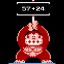Get a Score of 1000 Points in the easy Addition Exercise.

10
55.29% (43.3)
•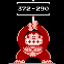Get a Score of 1000 Points in the easy Subtraction Exercise.

10
49.41% (49.8)
•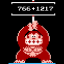Get a Score of 1000 Points in the medium Addition Exercise.

25
44.71% (56.7)
•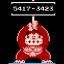Get a Score of 1000 Points in the medium Subtraction Exercise.

25
42.35% (60.8)
•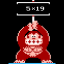Get a Score of 1000 Points in the medium Multiplication Exercise.

10
49.41% (49.8)
•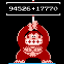Get a Score of 1000 Points in the hard Addition Exercise.

25
40.00% (70.5)
•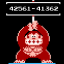Get a Score of 1000 Points in the hard Subtraction Exercise.

25
40.00% (70.5)
•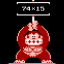Get a Score of 1000 Points in the hard Multiplication Exercise.

25
34.12% (154.1)
•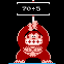Get a Score of 1000 Points in the Division Exercise.

25
38.82% (127.0)
•Win a round Calculate A.

10
97.65% (37.9)
•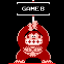Win a round Calculate B.

10
75.29% (40.5)